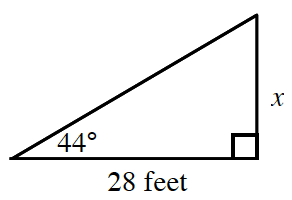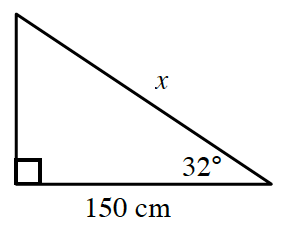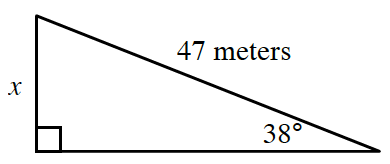### Home > CCA2 > Chapter 7 > Lesson 7.1.1 > Problem7-5

7-5.

In each of the following triangles, find the length of the side labeled $x$.

1.Which trigonometric relationship uses the opposite and adjacent sides?

$\tan (44^\circ)=\frac{x}{28}$

$27.04\ \text{feet}$

1.Identify the sides involved and choose the appropriate trigonometric relationship.

1.See part (b).